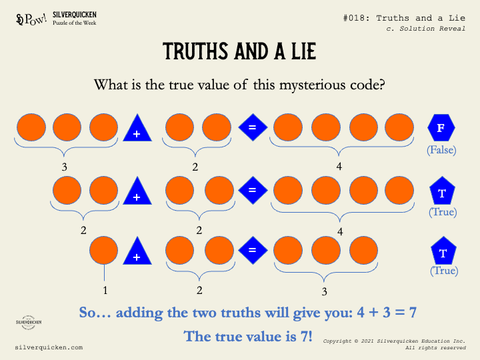# Week 18 - Solution: Truths and a Lie

Did you understand that this puzzle is a series of mathematical statements represented by symbols? The shapes and colors did, indeed, have different meanings!

- The orange circles each stand for the number "1";
- The blue triangle stands for “plus”;
- The blue diamond stands for “equals”;
- The pentagon stands for “true”; and
- The hexagon stands for “false”.

So if you put that all together, you have three mathematical statements, AND the note about whether they're true or false!

3 + 2 = 4 -- FALSE
2 + 2 = 4  -- TRUE!
1 + 2 = 3 -- TRUE!

So what's the true value of the code?

4 + 3 = 7!!!Hope you enjoyed this one. See you tomorrow for a new puzzle!